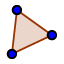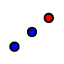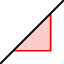# Module 10 Coordinate Proof Using Slope & Distance

Draw a quadrilateral using the polygon toolso that no two sides are congruent or parallel. Hint: choose even number coordinates to make the math easier.

## Coordinate Points

Write the four coordinate points with labels.

## Task 2 Calculate distance of AB

Calculate the distance from A to B. Use the example below to show the work. A (8, 1) B(2, 4) d(AB) = SQRT ( ( x₂ - x₁)^2 + ( y₂ -y₁)^2 ) d(AB) = SQRT ( ( 8 - 2 )^2 + ( 1 - 4)^2 ) d(AB) = SQRT ( (6)^2 + (-3)^2 ) d(AB) = SQRT ( 36 + 9 ) d(AB) = SQRT ( 45) = 6.71

## Find the perimeter.

Go back to the applet. Measure the distance for all four sides using thetool.

## Find the Perimeter

Find the perimeter. Use the example below to show your work. AB = 6.71, BC = 5, CD = 10, DA = 8 Perimeter = 6.71 + 5 + 10 + 8 = 29.71

## Find midpoint of AB

Find the mid point of AB. Use the example below to show your work. A (8, 1) B(2, 4) mp(AB) = (x₁+x₂)/2, (y₁+y₂)/2, (mp(AB) = (8+2)/2, (1 + 4 )/2 mp(AB) = (10/2, 5/2) mp(AB) = ((5,2.5)

Go back to applet and use the midpoint toolto find the midpoint of all four sides. Start with midpoint of AB then BC, CD and DA. Then use the polygon toolto create a quadrilateral using the midpoints as vertices EFGH.

## Coordinates of E and F?

What are the coordinates of E and F?

## Slope of EF

Find the slope of EF. Show all your work. Follow the example below. E ( 2 , 6) F ( 4 10) m = ( y₂ - y₁) / ( x₂ - x₁) m = (10 - 6 ) / ( 4 -2 ) m = 4 / 2 =2

Go back to the applet and use the slope toolto find the slope of all four sides of the inscribed quadrilateral.

## What type of quadrilateral?

What type of quadrilateral did you form connecting the midpoints.Give the reason why.

Use the figure below to find the area and perimeter and answer the following questions.

Describe how you would find the perimeter of the figure above. Use as much detail as possible. Examples " count spaces, use distance formula, use Pythagorean theorem......Go line by line.

What is the perimeter?

Describe your strategy for finding the area of the shape. How would you break it up? What would be the coordinate points of the new shapes created? Label everything.

What is the area of the shape?